Question

# 3. A firm in a perfectly competitive market will produce no output in the short run...

3. A firm in a perfectly competitive market will produce no output in the short run if the price is below \$18 but will produce if the price is above \$18. The smallest quantity they will produce in the short run is 8. Firms will earn 0 economic profit if the price is \$74 and its profit maximizing quantity is 12 at that price. The firm’s fixed cost is \$576. Assume the good can be produced in continuous quantities.

Draw a picture of the MC, ATC, and AVC for this firm specifically labeling the values for each of these costs at q = 12 and q = 8. Round any decimal answers to 1 place. The diagram does not need to be to scale but needs the curves to exhibit the correct qualities. (2 points)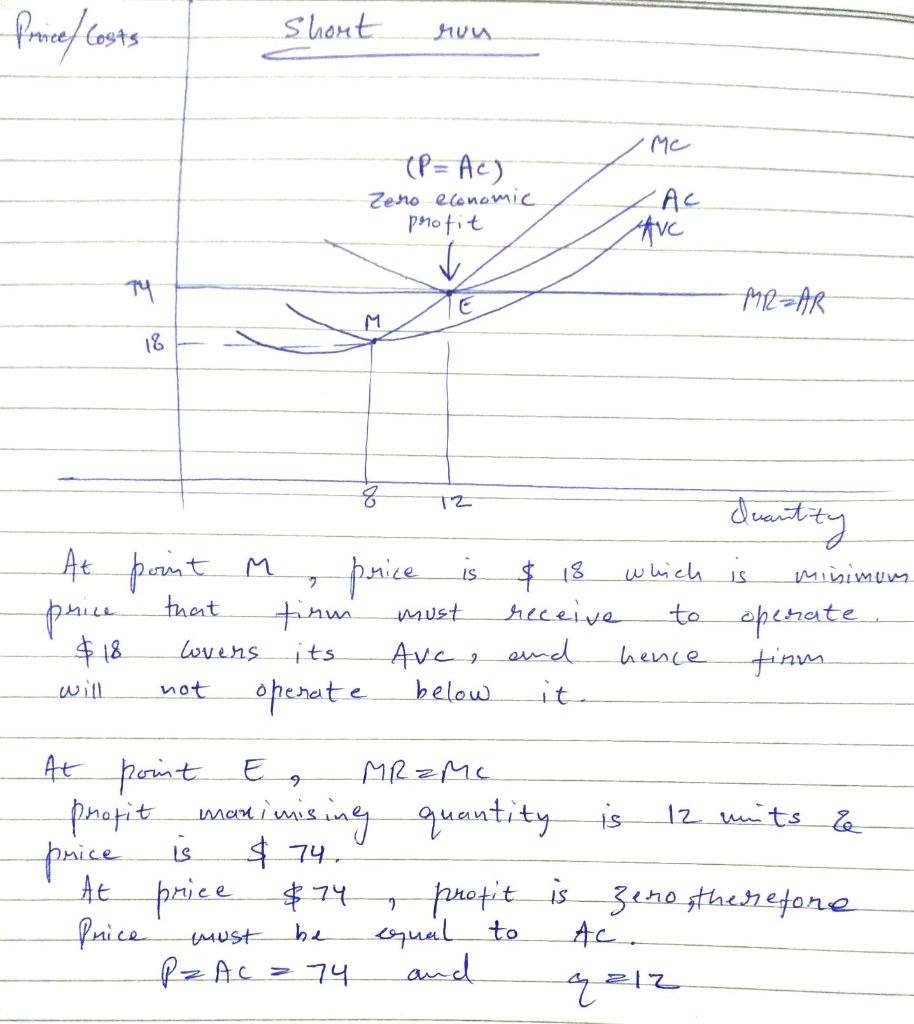#### Earn Coins

Coins can be redeemed for fabulous gifts.

Similar Homework Help Questions
• ### 5. Deriving the short-run supply curve Aa Aa Consider the perfectly competitive market for halogen ceiling...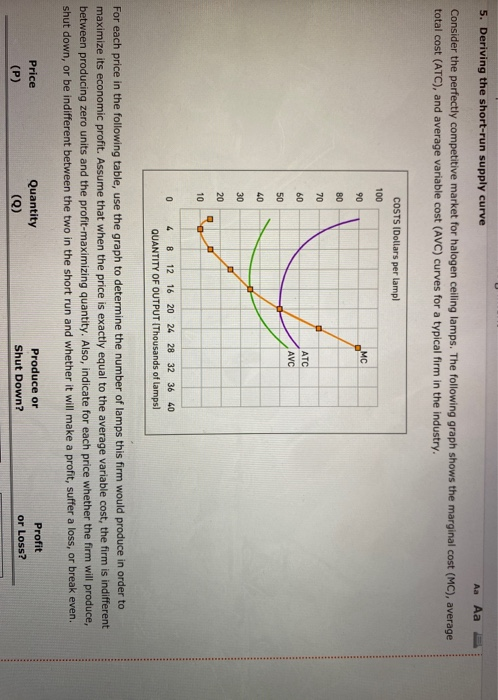5. Deriving the short-run supply curve Aa Aa Consider the perfectly competitive market for halogen ceiling lamps. The following graph shows the marginal cost (MC), average total cost (ATC), and average variable cost (AVC) curves for a typical firm in the industry. COSTS Dollars per lampl 100 MC 90 80 70 60 ATC AVC 50 40 30 20 10 0 4 8 12 16 20 24 28 32 36 40 QUANTITY OF OUTPUT (Thousands of lamps) For each price in...

• ### stions I through 10. Use the graph for a perfectly e graph for a perfectly competitive firm to answer questions I th...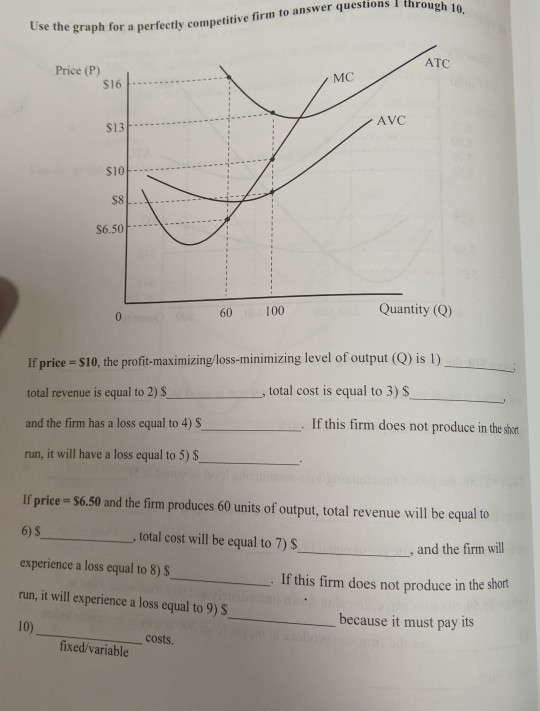stions I through 10. Use the graph for a perfectly e graph for a perfectly competitive firm to answer questions I throue ATC Price (P) S16 MC AVC \$13 \$10-- \$8 \$6.50 60 100 Quantity (Q) If price - \$10, the profit-maximizing/loss-minimizing level of output (Q) is 1) total revenue is equal to 2) total cost is equal to 3) \$ and the firm has a loss equal to 4) \$ If this firm does not produce in the short...

• ### This question is in regards to situations that might face a perfectly competitive firm. Draw two...

This question is in regards to situations that might face a perfectly competitive firm. Draw two graphs. On the first, show the short-run profit maximizing output of an individual firm earning an economic profit, including MR, MC, AVC, and ATC. On the second, show the short-run market equilibrium price and quantity. Explain how the industry supply curve and the market equilibrium price and quantity are determined.

• ### 4. Deriving the short-run supply curve Consider the perfectly competitive market for dress shirts. The following graph...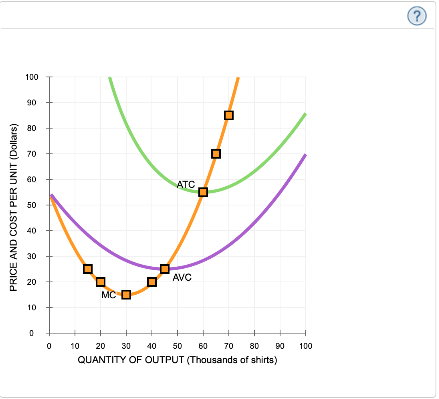4. Deriving the short-run supply curve Consider the perfectly competitive market for dress shirts. The following graph shows the marginal cost (MC), average total cost (ATC), and average variable cost (AVC) curves for a typical firm in the industry. that when the price is exactly equal to the average variable cost, the firm is indifferent between producing zero shirts and the profit-maximizing quantity. Also, indicate whether the firm will produce, shut down, or be indifferent between the two in the short run....

• ### "he graph below shows a profit-maximizing perfectly competitive firm operating in the short run. Which area...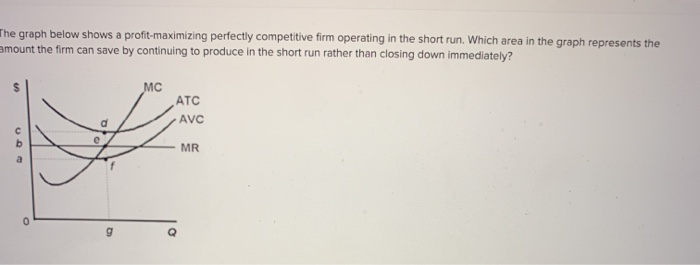"he graph below shows a profit-maximizing perfectly competitive firm operating in the short run. Which area in the graph represents the amount the firm can save by continuing to produce in the short run rather than closing down immediately? ATC AVC MR

• ### Sonya and Leah operate a small firm in a perfectly competitive market, the diagram illustrates its...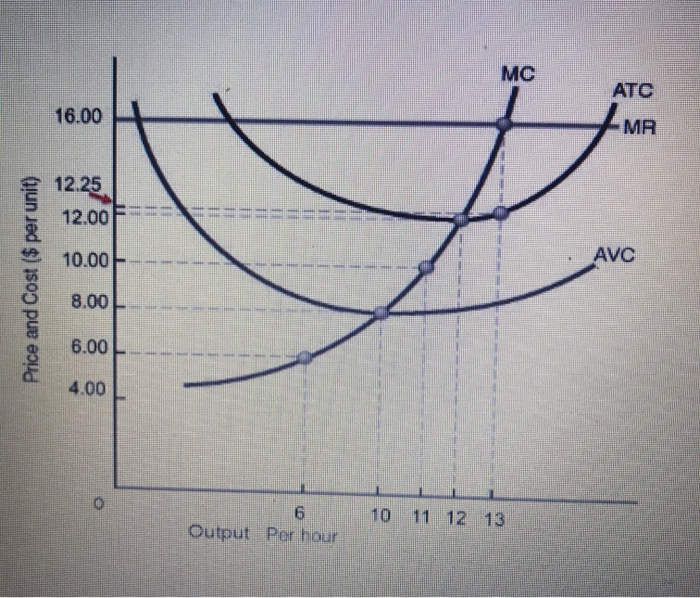Sonya and Leah operate a small firm in a perfectly competitive market, the diagram illustrates its MC, ATC, AVC and MR curves. 1. What is their current average revenue per unit? 2. What is their profit maximizing level of output and profit? 3. If the market clearing price drops to \$10.00 per unit, should they continue to produce in the short run if they wish to maximize their economic profits (or minimize its economic losses)? Explain. 4. What is their...

• ### The following graph shows the demand and cost curves for a perfectly competitive firm. The profit-maximizing...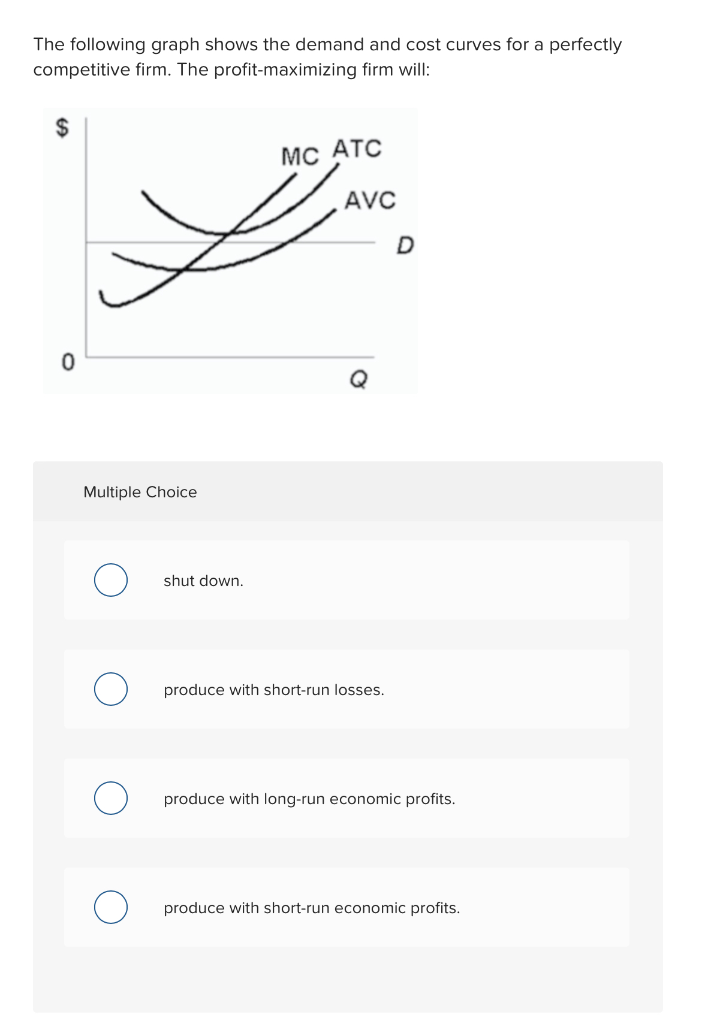The following graph shows the demand and cost curves for a perfectly competitive firm. The profit-maximizing firm will: MC ATC // AVC Multiple Choice shut down. ο produce with short-run losses. O produce with long-run economic profits. ο produce with short-run economic profits.

• ### Question 2: Consider a firm in a perfectly competitive market that should produce a positive quantity...

Question 2: Consider a firm in a perfectly competitive market that should produce a positive quantity in the short-run, but is still earning a loss. A.) Draw the cost curves (ATC, AVC, MC, MR) for the firm. Clearly label the quantity this firm would produce. Label the loss the firm would incur. B.) Briefly explain why the MR looks the way it does and why you placed it where you did on your diagram. (How do we determine MR for...

• ### 5. Deriving the short-run supply curve Consider the perfectly competitive market for dress shirts. The following...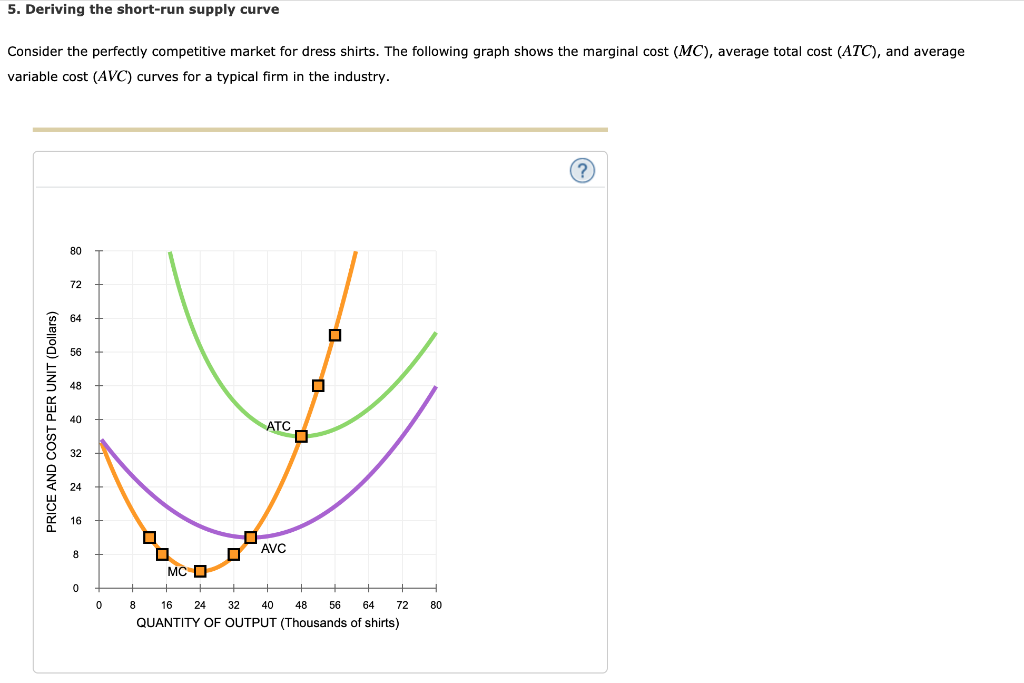5. Deriving the short-run supply curve Consider the perfectly competitive market for dress shirts. The following graph shows the marginal cost (MC), average total cost (ATC), and average variable cost (AVC) curves for a typical firm in the industry. ? 80 72 64 56 40 АТС AVC 8 МС О 0 8 16 24 32 40 48 56 64 72 80 QUANTITY OF OUTPUT (Thousands of shirts) PRICE AND COST PER UNIT (Dollars) For each price in the following table,...

• ### 1. Draw two graphs. On the first, show the short-run profit maximizing output of an individual...

1. Draw two graphs. On the first, show the short-run profit maximizing output of an individual firm earning an economic profit, including MR, MC, AVC, and ATC. On the second, show the short-run market equilibrium price and quantity. Explain how the industry supply curve and the market equilibrium price and quantity are determined. 2. What is the relationship between the price on the two graphs? Why does this relationship exist? 3. Explain why a firm in a perfectly competitive industry...